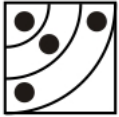# Mirror Image Questions for SSC Stenographer

0
393

## Mirror Image Questions for SSC Stenographer

SSC Stenographer Mirror Image Questions and Answers download PDF based on previous year question papers of SSC exam. Top – 10 Very important Mirror Image Questions for Stenographer.

Question 1: Choose the alternative which most closely resembles the mirror image of the given word when mirror is placed at XY line.

a)

b)

c)

d)

Question 2: Choose the alternative which most closely resembles the mirror image of the given word when mirror is placed at XY line.

a)

b)

c)

d)

Question 3: Choose the alternative which most closely resembles the mirror image of the given figure when mirror is placed at XY line.

a)

b)

c)

d)

Question 4: Choose the alternative which most closely resembles the mirror image of the given word when minor is placed at XY

a)

b)

c)

d)

Question 5: Select the answer figure that most closely resembles the mirror image of the given combination.

a)

b)

c)

d)

Question 6: If a mirror is placed on the line AB, then which of the answer figures is the right image of the given figure ?

a)

b)

c)

d)

Question 7: A mirror is placed on the line AB, then which of the answer figures is the right image of the given figure ?

a)

b)

c)

d)

Question 8:

a)

b)

c)

d)

Question 9: If a mirror is placed on the line AB, then which of the answer figures is the right image of the given figure ?

a)

b)

c)

d)

Question 10: If a mirror is placed on the line AB, then which of the answer figures is the right image of the given figure ?

a)

b)

c)

d)

SSC Stenographer Previous Papers

A horizontal mirror is placed, so the object on the top will appear at the bottom in reverse position and vice-versa.

So the three arcs facing bottom left will now face at top left, hence second option is the right image.

The mirror image :=> Ans – (B)

A horizontal mirror is placed, so the figure will be reversed, that is, the sector pointing downwards will face up and vice-versa, and thus the first and last options are not possible.

But the semi circle outside the quadrilateral will remain on the right side, as it is in the original figure, so the second option is also eliminated.

=> Ans – (C)

A horizontal mirror is placed, so the object on the top will appear at the bottom in reverse position and vice-versa.

So the vertical arrow in the middle facing downwards will now face upwards.

Also, in the question figure, the two circles at top right will now appear at bottom right side of the arrow, hence third option is the right image.

=> Ans – (C)